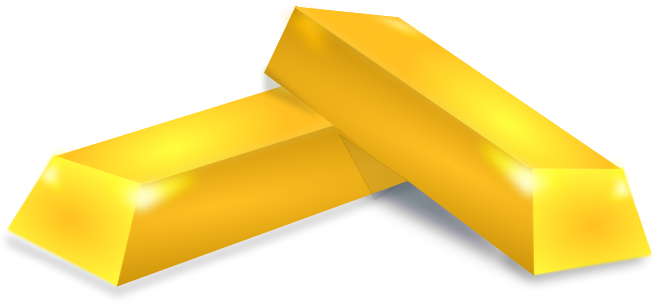# Two real numbers

## An Advanced Mathematics Lesson Starter Of The Day

Two numbers cubed add up to four,

Both real gold as you’ll see.

Their reciprocals sum to minus one,

What could those numbers be?Topics: Starter

How did you use this starter? Can you suggest how teachers could present or develop this resource? Do you have any comments? It is always useful to receive feedback and helps make this free resource even more useful for Maths teachers anywhere in the world.

Previous Day | This starter is for | Next Day

Clive, Bangkok

Saturday, March 7, 2015

"What a great starter puzzle this is. It revises so many concepts for IB students (Standard and Studies). This is how it went in my class today: The mission is to find the gold, it is in the labyrinth (of your mind!). The first obstacle is to know what kind of Maths topic this is: algebra? OK what kind of algebra is it? Simultaneous equations? Very good, can you write down those simultaneous equations? Can you solve those simultaneous equations? The simultaneous equation solver on the calculator is no good as it only solves for linear equations. How about drawing graphs and seeing where the graphs intersect? You will need to know how to rearrange the equations to make y the subject. Wow, what a surprising answer!"

Mollie Shoe, Georgia

Tuesday, May 5, 2020

"Hello, I've spent a very long time on this question and I still don't know the answer. How would you go about figuring it out?"

Pirenz, Philippines

Wednesday, January 13, 2021

"Let x and y represent the two real numbers.
1/x + 1/y = -1 {Second Given}
y + x = -xy {Multiply by xy}
Let u equal the value of x + y and -xy.
Hence:
4 = x^3 + y^3 {Given}
= (x + y) (x^2 - xy + y^2) {Factorize}
= (x + y) (x^2 + 2xy + y^2 - 3xy) {Rewrite -xy as 2xy - 3xy}
= (x + y) (x + y)^2 + 3(-xy) {Factor x^2 + 2xy + y^2}
= u (u^2 + 3u) {Substitute u = x + y and u = -xy}
= u^3 + 3u^2 {Expand}
So:
u^3 + 3u^2 - 4 = 0
(u - 1)(u + 2)(u + 2) = 0 {Factorize}
Thus, u = 1 or u = -2.
CASE I: u = -2
By definition, this means that x + y = -2 and -xy = -2
So, x + y = -2 and xy = 2
Hence, x ( -2 - x ) = 2 which means 0 = x^2 + 2x + 2.
But, x^2 + 2x + 2 is always at least 1 if x is a real number
So, this case provides no real solutions
CASE II: u = 1
By definition, this means that x + y = 1 and -xy = 1
So, x + y = 1 and xy = -1
Hence, x ( 1 - x ) = -1 which means 0 = x^2 - x - 1
x = (1 + sqrt(5))/2 and y = (1 - sqrt(5))/2
OR
x = (1 - sqrt(5))/2 and y = (1 + sqrt(5))/2
So, the numbers are (1 + sqrt(5))/2 and (1 - sqrt(5))/2
Note that (1 + sqrt(5))/2 is the golden ratio this is exactly why the second line said "Both real GOLD as you'll see".
Good one, Transum! :)."

How did you use this resource? Can you suggest how teachers could present, adapt or develop it? Do you have any comments? It is always useful to receive feedback and helps make this free resource even more useful for Maths teachers anywhere in the world. Click here to enter your comments.

Your access to the majority of the Transum resources continues to be free but you can help support the continued growth of the website by doing your Amazon shopping using the links on this page. Below is an Amazon link. As an Amazon Associate I earn a small amount from qualifying purchases which helps pay for the upkeep of this website.

Educational Technology on AmazonFor All: